# Fitting growth models in biogrowth

library(biogrowth)
library(tidyverse)

In most situations, the model parameters of growth models cannot be known beforehand. Instead, they must be estimated from experimental data. For this reason, model fitting is a central part of modeling the growth of populations. The biogrowth package can do four types of model fitting:

• fitting primary growth models
• fitting both primary and secondary models from a single experiment under dynamic environmental conditions
• fitting both primary and secondary models from multiple experiments (often called “global fitting”)
• fitting secondary models to a dataset of growth rates

This vignette describes the two functions that biogrowth implements for this: fit_growth() and fit_secondary_growth().

## Fitting growth models with fit_growth()

Since version 1.0.0, biogrowth includes the fit_growth() function that provides a top-level interface for fitting primary (and secondary) growth models from experimental data. This function includes several arguments to describe the fitting approach, the model equations and the experimental data, as well as other factors of the model fitting.

The type of model to fit is defined in the environment argument. If environment="constant" (default), the function only fits a primary growth model to the data. On the other hand, if environment="dynamic", the model fits both primary and secondary models according to the gamma approach. In this case, the function can fit the growth model either to a single growth experiment or to several ones (with different environmental conditions). This behavior is controlled by argument approach.

Once the fitting approach has been defined, the model equations are defined through the model_keys argument, and the experimental data through fit_data. Models fitted under dynamic environmental conditions are defined through env_conditions.

Model fitting is done through the FME package. This package includes two functions for model fitting: modFit() that uses (non-linear) regression, and modMCMC() that uses an adaptive Monte Carlo algorithm. The function fit_growth() allows the selection of a fitting approach using the algorithm argument. If the MC algorithm is selected, the number of iterations is defined through niter. Both algorithms need initial guesses for every model parameter. These can be defined through the start argument. The biogrowth package includes several functions to aid in the definition of starting guesses of the model parameters, which will be described below. Furthermore, fit_growth() allows fixing any model parameter with the known argument. Lastly, additional arguments can be passed to either modFit() or modMCMC() with the ... argument.

Apart from that, the function includes several checks of the model definition. These can be turned off setting check=FALSE.

Both predict_growth() and fit_growth() can make the calculations in different log-bases for both the population size and parameter $$\mu$$ using the arguments logbase_mu and logbase_logN. The reader is referred to the specific vignette dedicated to this topic for details on the units definition and the interpretation.

Finally, the formula argument maps the elapsed time and the population size to the column names in the data. By default, formula = logN ~ time, meaning that the elapsed time is named time and the population size is named logN in the data.

The following sections provide additional details on the use of fit_growth() for different types of model fitting.

### Fitting primary growth models

The most simple approach implemented in fit_growth() is fitting primary growth models to experimental data that includes the variation of a population during an experiment. This behaviour is defined when environment="constant". In this case, fit_growth() requires the definition of (at least) 4 arguments:

• fit_data to pass the experimental data
• model_keys defining the model equation
• start setting the initial values for the model parameters
• known defining the fixed model parameters

The experimental data must be defined as a tibble (or data frame). It must include two columns: one defining the elapsed time (by default, named time) and a second one defining the logarithm of the population size (by default, named logN). Note that the default names can be changed using the formula argument. In this example, we will define a tibble by hand.


my_data <- data.frame(time = c(0, 10, 15, 20, 25, 30, 35, 40, 45, 50),
logN = c(1.2, 1, 1.7, 1.9, 2.3, 3.1, 3.3, 3.8, 4.3, 4.1)
)

ggplot(my_data) + geom_point(aes(x = time, y = logN))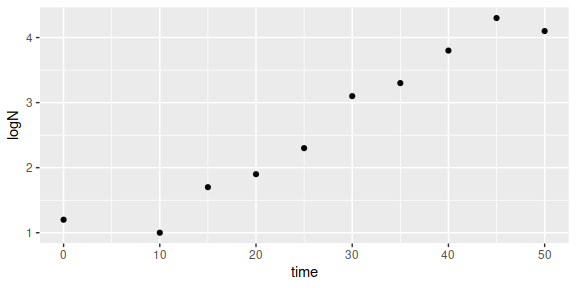Note that, by default, the population size is interpreted in log10 scale. The base of the logarithm can be modified using the logbase_logN argument. Please check the vignette dedicated to the topic for further details.

The next step in model fitting is the definition of the growth model to fit to the data using the model_keys argument. This argument is a named list assigning model equations to the different models. In the case when environment="constant", the functions only fits a primary model. Therefore, model_keys must have a unique entry named “primary” with the model key of a primary model. A list of valid keys can be retrieved calling primary_model_data() without any argument.

primary_model_data()
#>  "modGompertz" "Baranyi"     "Trilinear"   "Logistic"    "Richards"

In this example, we will use the Baranyi growth model.

models <- list(primary = "Baranyi")

In the case of primary model fitting, fit_growth() uses non-linear regression through modFit(). This algorithm requires the definition of initial guesses for every model parameter. This is done in fit_growth() through the argument start, which is a named numeric vector (or list). The names of the vector must be parameter keys defined within biogrowth. These can be retrieved (as well as additional model meta-data) by passing a model key to primary_model_data().

primary_model_data("Baranyi")$pars #>  "logN0" "logNmax" "mu" "lambda" Therefore, the Baranyi model requires the definition of four model parameters. For a description of the model equations and the interpretation of the model parameters, please check the specific package vignette. The biogrowth package includes several functions to aid in the definition of initial guesses for the model parameters. The function check_growth_guess() takes three arguments: • fit_data defining the experimental data as in fit_growth() • model_name, a character vector of length one defining the primary model (as per primary_model_data()) • guess, a numeric vector defining the initial guess as in fit_growth() • environment, describing the type of environmental conditions ("constant" (default) or "dynamic") • env_conditions defining the variation of the environmental conditions (only if environment="dynamic") Then, the function creates a plot comparing the experimental data against the initial guess. start <- c(logNmax = 6, lambda = 25, logN0 = 2, mu = .2) # Initial guess check_growth_guess( my_data, models, start )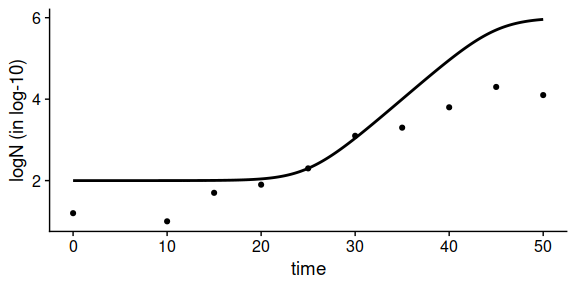In this case, the plot shows that the initial guess is moderately far from the experimental data. For instance, the values of logN0 and logNmax are too high. These could potentially cause some convergence issues. Note that this function can use different log-scales using logbase_mu or logbase_logN. In order to facilitate the definition of initial guesses, biogrowth includes make_guess_primary(). This function applies some heuristic rules to define guesses for the initial model parameters. It takes two arguments: • fit_data that passes the experimental data as described above • primary_model that defines the growth model Furthermore, the function includes a logbase_mu argument to define the logbase of parameter $$\mu$$. Calling this function with our data and the Baranyi model returns a named vector with an initial guess of the model parameters. auto_guess <- make_guess_primary(my_data, "Baranyi") print(auto_guess) #> logN0 mu lambda logNmax #> 1.20000000 0.08857143 10.00000000 4.30000000 We can now use check_growth_guess() to assess the quality of the initial guess  check_growth_guess( my_data, models, auto_guess )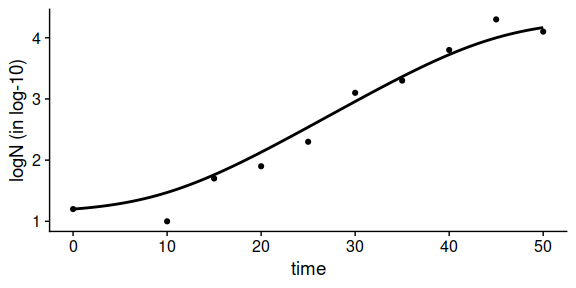From the plot, we can see that the initial guess is already quite close to the experimental data. The last argument to be defined is known, which allows fixing any model parameter to a given value. This argument is also a named list with the same conventions as start. In a first example, we will define it as an empty vector, indicating that no model parameter is fixed (i.e. every one is estimated from the data). known <- c() Then, we can call fit_growth() with these arguments. primary_fit <- fit_growth(my_data, models, auto_guess, known, environment = "constant", ) The function returns an instance of GrowthFit. It includes several S3 methods to facilitate the interpretation of the results of the model fit. For instance, the print() method provides a quick view of the model fitted: print(primary_fit) #> Primary growth model fitted to data #> #> Growth model: Baranyi #> #> Estimated parameters: #> logN0 mu lambda logNmax #> 1.0987559 0.1104077 13.2555216 4.2695511 #> #> Fixed parameters: #> NULL #> #> Parameter mu defined in log-10 scale #> Population size defined in log-10 scale The statistical summary of the fit can be retrieved using the summary() method. summary(primary_fit) #> #> Parameters: #> Estimate Std. Error t value Pr(>|t|) #> logN0 1.09876 0.16566 6.633 0.000566 *** #> mu 0.11041 0.01511 7.306 0.000336 *** #> lambda 13.25552 3.09831 4.278 0.005215 ** #> logNmax 4.26955 0.20220 21.116 7.35e-07 *** #> --- #> Signif. codes: 0 '***' 0.001 '**' 0.01 '*' 0.05 '.' 0.1 ' ' 1 #> #> Residual standard error: 0.1925 on 6 degrees of freedom #> #> Parameter correlation: #> logN0 mu lambda logNmax #> logN0 1.0000 0.3849 0.7906 -0.1485 #> mu 0.3849 1.0000 0.8020 -0.5394 #> lambda 0.7906 0.8020 1.0000 -0.3253 #> logNmax -0.1485 -0.5394 -0.3253 1.0000 And the plot() method compares the model fitted against the experimental data. This function takes additional arguments that enable editing several aesthetics of the plot. For a complete list, please check the help page of GrowthFit. Note that this method returns an instance of ggplot, so it can be edited using additional layers. plot(primary_fit, line_col = "red", point_shape = 1)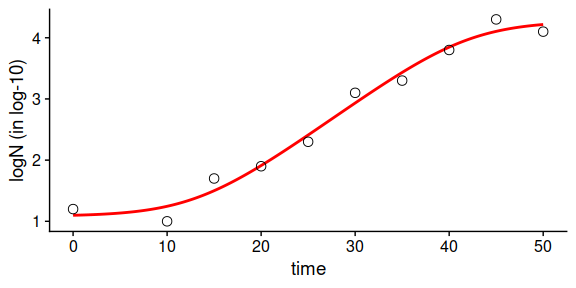The instance of GrowthFit includes additional methods to inspect and use the fitted model (predict, vcov, AIC…). For a complete list, please check its help page. Moreover, this instance is a subclass of list, making it easy to access several aspects of the data. Namely, it has the following entries: • data with the experimental data • start initial values of the model parameters • known fixed model parameters • best_prediction an instance of GrowthPrediction with the fitted curve • logbase_mu the logbase for $$\mu$$ • logbase_logN the logbase for the population size • environment the type of environment ("constant" for this type of fit) • algorithm the fitting algorithm ("regression" for this type of fit) • primary_model the primary model fitted • fit_results an instance of modFit with the fitted model As was mentioned above, the known argument allows fixing any model parameter to a given value. It must be a named vector with the same conventions as for the initial values. For instance, we can fix the maximum population size to 4. known <- c(logNmax = 4) If this argument was passed to fit_growth() together with auto_guess, the function would return a warning (as long as check=TRUE). The reason for this is that logNmax is defined both as an initial guess (i.e. a parameter to fit) and a fixed parameter. Therefore, the initial guess must be modified, including only the parameters to fit (i.e. logN0, mu and lambda) new_guess <- auto_guess[c("logN0", "mu", "lambda")] new_fit <- fit_growth(my_data, models, new_guess, known, environment = "constant", ) The print method of the new_fit reflects the fact that logNmax was now fixed. new_fit #> Primary growth model fitted to data #> #> Growth model: Baranyi #> #> Estimated parameters: #> logN0 mu lambda #> 1.1178327 0.1194789 14.0961391 #> #> Fixed parameters: #> logNmax #> 4 #> #> Parameter mu defined in log-10 scale #> Population size defined in log-10 scale And the summary method only includes the statistical information for the fitted parameters. summary(new_fit) #> #> Parameters: #> Estimate Std. Error t value Pr(>|t|) #> logN0 1.11783 0.17671 6.326 0.000394 *** #> mu 0.11948 0.01813 6.590 0.000307 *** #> lambda 14.09614 3.14958 4.476 0.002882 ** #> --- #> Signif. codes: 0 '***' 0.001 '**' 0.01 '*' 0.05 '.' 0.1 ' ' 1 #> #> Residual standard error: 0.2129 on 7 degrees of freedom #> #> Parameter correlation: #> logN0 mu lambda #> logN0 1.0000 0.3828 0.7810 #> mu 0.3828 1.0000 0.8007 #> lambda 0.7810 0.8007 1.0000 Another relevant function included in biogrowth is time_to_size. This function takes two arguments: • model an instance of GrowthFit (or other object returned by fit_growth()) • size a target population size (in log units) Then, it calculates the elapsed time required to reach the given size time_to_size(primary_fit, 2) #>  20.93122 If the target population size is not reached within the fitted curve, the function returns NA. time_to_size(primary_fit, 8) #>  NA This function can take two additional arguments. The argument logbase_logN allows defining size in different log-bases. By default, this function assumes the same base as the one used in the instance of GrowthFit. The second argument is type. By default, type="discrete", indicating that the function calculates a single value of the elapsed time required to reach the target population size. Setting type = "distribution" accounts for parameter uncertainty and calculates a distribution for the elapsed time. This is described in detail in the vignette about including uncertainty in growth predictions. ### Fitting both primary and secondar models from a single experiment under dynamic environnental conditions The fit_growth() function can also be used to fit a growth model based on both primary and secondary models to a set of data gathered under dynamic experimental conditions. This behavior is triggered by setting environment="dynamic". Then, fit_growth() supports two fitting approaches. The first one assumes that every data point was gathered (at different time points of) one or more growth experiments under a single, dynamic environmental profile. This method is set up setting approach="single". The second one, triggered when approach="global" considers that different experiments could have different (dynamic) environmental conditions. This section describes the former approach, whereas the latter will be described in the following section. In this case, when environment="dynamic" and approach="single", the function requires the definition of the following arguments: • fit_data to pass the experimental data • model_keys defining the model equation • start setting the initial values for the model parameters • known defining the fixed model parameters • algorithm selecting either regression or an Adaptive Monte Carlo algorithm • env_conditions describing the variation of the environmental conditions during the experiment • niter: number of iterations of the MC algorithm (only if algorithm="MCMC") The arguments env_conditions and fit_data are tibbles (or data frames) that describe, respectively, the experimental design and the observations. The biogrowth package includes two example datasets to illustrate the input requirements for this function. data("example_dynamic_growth") data("example_env_conditions") The tibble passed to the argument env_conditions must have a column defining the elapsed time (time by default) and as many additional columns as environmental factors. The fit_growth() function is totally flexible with respect to the number of factors or the way they are named. The only requirement is that the definition of every argument is consistent. In the case of example_env_conditions, this dataset considers two factors: temperature and water activity (aw). head(example_env_conditions) #> # A tibble: 3 × 3 #> time temperature aw #> <dbl> <dbl> <dbl> #> 1 0 20 0.99 #> 2 5 30 0.95 #> 3 15 35 0.9 Note that for the calculations this function joins the data points by linear interpolation, as shown in this plot: ggplot(example_env_conditions, aes(x = time, y = temperature)) + geom_line() + geom_point()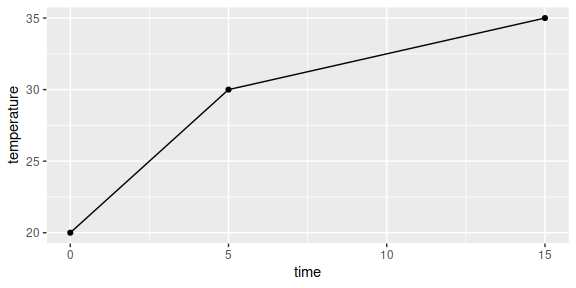The tibble passed to the argument fit_data must have one column defining the elapsed time (time by default) and one defining the logarithm of the population size (logN by default). Different column names can be defined using the formula argument. For instance, formula=log_size ~ Time would mean that the elapsed time is called “Time” and the logarithm of the population size is called “log_size”. Note that the name of the column describing the elapsed time in fit_data must be identical to the one in env_conditions. head(example_dynamic_growth) #> # A tibble: 6 × 2 #> time logN #> <dbl> <dbl> #> 1 0 0.0670 #> 2 0.517 -0.00463 #> 3 1.03 -0.0980 #> 4 1.55 -0.0986 #> 5 2.07 0.111 #> 6 2.59 -0.0465 By default, the calculations are done considering that logN is defined in log10 scale. Nonetheless, this can be modified with the argument logbase_logN. Please check the vignette on the topic for additional details. The type of secondary model for each environmental factor is defined by the argument model_names. This argument is a named vector where the names refer to the environmental factor and the value to the type of model. Supported models can be retrieved using secondary_model_data(). secondary_model_data() #>  "CPM" "Zwietering" "fullRatkowsky" In this example we will use cardinal models for water activity and a Zwietering-type model for temperature. Note that the names of this vector must be identical to the columns in env_conditions. sec_model_names <- list(temperature = "Zwietering", aw = "CPM") This modelling approach implements two algorithms for model fitting: non-linear regression (FME::modFit()) and an adaptive Monte Carlo algorithm (FME:modMCMC()). This can be selected through the algorithm argument. If algorithm="regression" (default), the fitting is done with FME:modFit(). If algorithm="MCMC", the fitting is done with FME::modMCMC(). In this vignette, we focus on the former. For a description on MCMC fitting, please check the vignette on modelling growth including uncertainty. Regardless of the fitting algorithm, fit_growth() enables to fit or fix any model parameter. This distinction is made using the arguments known (fixed parameters) and start (fitted parameters). Note that every parameter of the primary and secondary model must be in either of these arguments without duplication. This function uses the Baranyi primary model. It has two variables that need initial values (N0 and Q0) and one primary model parameter (Nmax). The specific growth rate is described using the gamma concept. This requires the definition of its value under optimal conditions (mu_opt) as well as the cardinal parameters for each environmental factor. They must be defined as factor+_+parameter name. For instance, the minimum water activity for growth must be written as aw_xmin. In this example we will consider the model parameters of the primary model as known. For the secondary model for water activity, we will only consider the optimum value for growth as unknown. Finally, for the effect of temperature, we will consider the order and xmax are known: known_pars <- c(Nmax = 1e4, # Primary model N0 = 1e0, Q0 = 1e-3, # Initial values of the primary model mu_opt = 4, # mu_opt of the gamma model temperature_n = 1, # Secondary model for temperature aw_xmax = 1, aw_xmin = .9, aw_n = 1 # Secondary model for water activity ) Then, the remaining model parameters must be defined in start. Due to the use of non-linear regression for model fitting, it is required to define initial values for these parameters. In order to ease the definition of initial guesses for these parameters, biogrowth includes the function check_growth_guess(). As already mentioned, it takes five arguments: • fit_data defining the experimental data as in fit_growth() • model_name, a character vector of length one defining the primary model (as per primary_model_data()) • guess, a numeric vector defining the initial guess as in fit_growth() • environment, describing the type of environmental conditions ("constant" (default) or "dynamic") • env_conditions defining the variation of the environmental conditions (only if environment="dynamic") All these arguments follow the same conventions as in fit_growth(). Furthermore, the function includes additional arguments to define the log-base of $$\mu$$ (logbase_mu) and to define the column names for the population size and the elapsed time (formula). Then, we can define an initial guess of the model parameters my_start <- c(temperature_xmin = 25, temperature_xopt = 35, aw_xopt = .95) And pass it to check_growth_guess(), together with the values of the fixed parameters check_growth_guess( example_dynamic_growth, sec_model_names, c(my_start, known_pars), environment = "dynamic", env_conditions = example_env_conditions )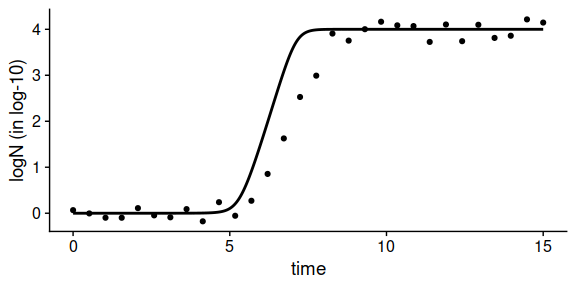This plot shows that the initial guess of the model parameters is reasonably close to the experimental data. Then, once every argument has been defined, we can call fit_growth()  my_dyna_fit <- fit_growth(example_dynamic_growth, sec_model_names, my_start, known_pars, environment = "dynamic", env_conditions = example_env_conditions )  Again, fit_growth() returns an instance of GrowthFit with the same S3 methods as for models fitted under constant environmental conditions. print(my_dyna_fit) #> Growth model fitted to data gathered under dynamic environmental conditions using regression #> #> Environmental factors included: temperature, aw #> #> Secondary model for temperature: Zwietering #> Secondary model for aw: CPM #> #> Parameter estimates: #> temperature_xmin temperature_xopt aw_xopt #> 25.7920469 32.4099118 0.9886544 #> #> Fixed parameters: #> Nmax N0 Q0 mu_opt temperature_n #> 1e+04 1e+00 1e-03 4e+00 1e+00 #> aw_xmax aw_xmin aw_n #> 1e+00 9e-01 1e+00 #> Parameter mu defined in log-10 scale #> Population size defined in log-10 scale summary(my_dyna_fit) #> #> Parameters: #> Estimate Std. Error t value Pr(>|t|) #> temperature_xmin 25.79205 0.54978 46.91 < 2e-16 *** #> temperature_xopt 32.40991 2.45444 13.21 2.7e-13 *** #> aw_xopt 0.98865 0.01823 54.24 < 2e-16 *** #> --- #> Signif. codes: 0 '***' 0.001 '**' 0.01 '*' 0.05 '.' 0.1 ' ' 1 #> #> Residual standard error: 0.1478 on 27 degrees of freedom #> #> Parameter correlation: #> temperature_xmin temperature_xopt aw_xopt #> temperature_xmin 1.00000 -0.2999 0.06465 #> temperature_xopt -0.29985 1.0000 -0.97056 #> aw_xopt 0.06465 -0.9706 1.00000 Nonetheless, the plot() method can include the variation of one environmental factor alongside the growth curve. This is done by passing the name of an environmental factor to the add_factor argument. Note that this name must be identical to the ones used for model definition. plot(my_dyna_fit, add_factor = "temperature")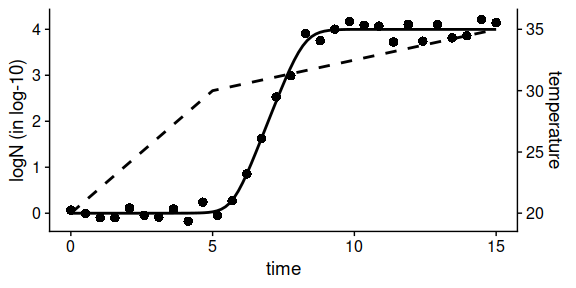Again, the time_to_size() function can be used to calculate the time required to reach a given population size (in log units): time_to_size(my_dyna_fit, 3) #>  7.629777 ### Fitting both primary and secondary models from several experiments (global fitting) As mentioned above, fit_growth() can also fit a unique growth model to a set of experimental data including the results of growth experiments under different (dynamic) environmental conditions. This is triggered passing approach="global". In this case, the function takes the same arguments as for approach="single". • fit_data to pass the experimental data • model_keys defining the model equation • start setting the initial values for the model parameters • known defining the fixed model parameters • algorithm selecting either regression or an Adaptive Monte Carlo algorithm • env_conditions describing the variation of the environmental conditions during the experiment • niter: number of iterations of the MC algorithm (only if algorithm="MCMC") However, the conventions for the definition of fit_data and env_conditions change when approach="global". Instead of being defined as a tibble (or data.frame), this information must be defined as a (named) list with as many elements as experiments. Then, each entry describes the particular information for each experiment. The biogrowth package includes the multiple_counts dataset as an example of this. It is a list of two elements named exp1 and exp2. Then, each one of them is a tibble (it could also be a data.frame) describing the experimental observations for each experiment following the same conventions as when approach="single" data("multiple_counts") names(multiple_counts) #>  "exp1" "exp2" head(multiple_counts[]) #> time logN #> 1 0.000000 -0.20801574 #> 2 1.666667 -0.03630986 #> 3 3.333333 -0.29846914 #> 4 5.000000 0.35029686 #> 5 6.666667 0.14326140 #> 6 8.333333 -0.40357904 In a similar way, the variation of the experimental conditions during the experiment are also described as a (named) list. This list should have the same length (and names) as the experimental data, so each growth experiment is mapped to an environmental profile. The conditions during each experiment are described following the same conventions as when algorithm="single". That is, using a tibble (or data.frame) with one column describing the elapsed time and as many additional columns as environmental factors considered in the model. Note that, although the function admits any number of environmental conditions, these must be presnet in each experiment. The multiple_conditions dataset included in the package is a convenient example of this format. It includes the results of two experiments, paired with multiple_counts, that consider two environmental factors: temperature and pH. data("multiple_conditions") names(multiple_conditions) #>  "exp1" "exp2" head(multiple_conditions[]) #> time temperature pH #> 1 0 20 6.5 #> 2 15 30 7.0 #> 3 40 40 6.5 The model equations, the initial guesses of the model parameters and the fixed parameters are defined in exactly the same way as when approach="single" sec_models <- list(temperature = "CPM", pH = "CPM") ## Any model parameter (of the primary or secondary models) can be fixed known_pars <- list(Nmax = 1e8, N0 = 1e0, Q0 = 1e-3, temperature_n = 2, temperature_xmin = 20, temperature_xmax = 35, pH_n = 2, pH_xmin = 5.5, pH_xmax = 7.5, pH_xopt = 6.5, temperature_xopt = 30) ## The rest, need initial guesses my_start <- list(mu_opt = .8) Again, check_growth_guess() can be used to assess the quality of the initial guess of the model parameters. check_growth_guess( multiple_counts, sec_models, c(my_start, known_pars), environment = "dynamic", env_conditions = multiple_conditions, approach = "global" )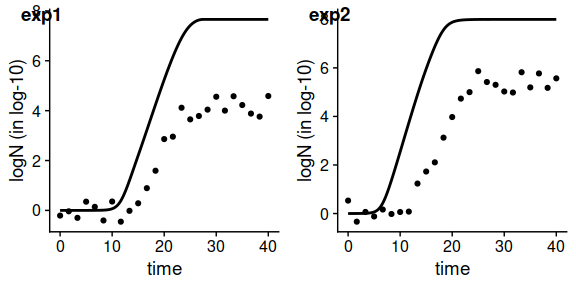Considering that the initial guess is relatively close to the observations, once all the arguments have been defined, we can call fit_growth() global_fit <- fit_growth(multiple_counts, sec_models, my_start, known_pars, environment = "dynamic", algorithm = "regression", approach = "global", env_conditions = multiple_conditions )  In this case, the function returns an instance of GlobalGrowthFit. The print method provides a summary of the model fitted. print(global_fit) #> Growth model fitted to data following a global approach conditions using regression #> #> Number of experiments: 2 #> #> Environmental factors included: temperature, pH #> #> Secondary model for temperature: CPM #> Secondary model for pH: CPM #> #> Parameter estimates: #> mu_opt #> 0.5097542 #> #> Fixed parameters: #> Nmax N0 Q0 temperature_n #> 1.0e+08 1.0e+00 1.0e-03 2.0e+00 #> temperature_xmin temperature_xmax pH_n pH_xmin #> 2.0e+01 3.5e+01 2.0e+00 5.5e+00 #> pH_xmax pH_xopt temperature_xopt #> 7.5e+00 6.5e+00 3.0e+01 #> Parameter mu defined in log-10 scale #> Population size defined in log-10 scale A more detailed output of the fitted model can be retrieved by summary() summary(global_fit) #> #> Parameters: #> Estimate Std. Error t value Pr(>|t|) #> mu_opt 0.509754 0.005812 87.7 <2e-16 *** #> --- #> Signif. codes: 0 '***' 0.001 '**' 0.01 '*' 0.05 '.' 0.1 ' ' 1 #> #> Residual standard error: 0.463 on 49 degrees of freedom #> #> Parameter correlation: #> mu_opt #> mu_opt 1 In this case, plot() shows several subplots, one for each experiment plot(global_fit)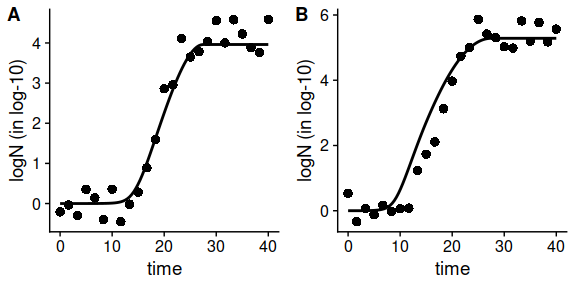As well as before, the plot can include the variation of any environmental factor alongside the growth curve, by passing the name of an environmental factor to add_factor. This function includes additional arguments for editing other aesthetics of the plot. For a complete list of options, please check the help page of GlobalGrowthFit. plot(global_fit, add_factor = "temperature", label_y2 = "Temperature (ºC)", line_col2 = "green", line_type2 = "dotted")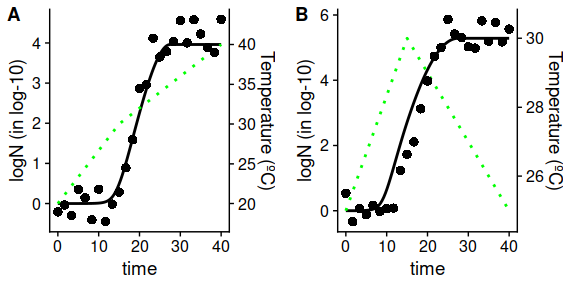Again, the time_to_size() function can be used to estimate the elapsed time required to reach a given population size (in log units). In this case, the function returns a named vector, with the elapsed time required to reach the target population size for each experiment time_to_size(global_fit, 3) #> exp1 exp2 #> 22.16405 15.76971 If the target population size was not reached for any of the experiments, the function returns NA time_to_size(global_fit, 5) #> exp1 exp2 #> NA 23.44123 ## Fitting secondary growth models with fit_secondary_growth() The other modeling approach included in biogrowth is fitting secondary growth models to a dataset comprising several growth rates estimated from various growth experiments (i.e. several values of $$\mu$$). Because the hypotheses under this approach are very different from those used for fitting primary models and primary & secondary models, this approach is implemented in a separate function: fit_secondary_growth(). This function has 8 arguments: • fit_data: data used for the fit. • starting_point: initial value of the model parameters • known_pars: model parameters fixed (not fitted to the data). • sec_model_names: type of secondary model for each environmental factor. • transformation: transformation of the growth rate for the fit (square root by default). • ...: additional arguments passed to modFit(). • check: whether to do some validity checks of the model parameters (TRUE by default). • formula: a formula defining the column name defining the growth rate. The fit_data argument must be a tibble containing the growth rates observed in several experiments under static environmental conditions. It must have one column describing the observed growth rate. Then, it must have as many additional columns as environmental factors included in the experiment. By default, the column describing the growth rate must be named mu. This can be changed using the formula argument, which is a one-sided formula, where the left hand side defines the column name. The biogrowth package includes the dataset example_cardinal to illustrate the data used by this function. It represents the specific growth rate (in log10 CFU/h) observed in several growth experiments under static environmental conditions, where each row represent one experiment. In this simulated dataset, two environmental factors were considered: temperature and pH. data("example_cardinal") head(example_cardinal) #> temperature pH mu #> 1 0.000000 5 9.768505e-04 #> 2 5.714286 5 2.624919e-03 #> 3 11.428571 5 0.000000e+00 #> 4 17.142857 5 1.530706e-04 #> 5 22.857143 5 2.301817e-05 #> 6 28.571429 5 3.895598e-04 In the example dataset, the series of experiments considered two environmental conditions: temperature and pH. Nonetheless, the fit_secondary_growth() function is entirely flexible with respect to the number of factors and their names. The only restriction is that the definition of the columns of the dataset and the secondary models is consistent. The type of secondary model to use for each environmental factor is defined in the sec_model_names argument. It is a named vector whose names are the environmental factors and whose values define the model to use. The model keys implemented in biogrowth can be retrieved using secondary_model_data(). secondary_model_data() #>  "CPM" "Zwietering" "fullRatkowsky" For this example, we will use a CPM for pH and an Zwietering model for temperature (this decision is not based on any scientific argument, just as demonstration of the functions in the package). Note that the names of the vector are identical to the column names of fit_data. sec_model_names <- c(temperature = "Zwietering", pH = "CPM") The fit_secondary_growth() function estimates the values of the cardinal parameters, as well as the growth rate under optimal conditions using the modFit function from FME, which uses non-linear regression. This algorithm requires the definition of initial guesses for every parameter estimate. These can be defined based on experience or exploratory analysis. In order to facilitate this definition, biogrowth includes the function make_guess_secondary(). This function makes a guess of the model parameters based on basic heuristic rules. It takes two arguments: • fit_data the data for the fit • sec_model_names a named vector assigning models for each environmental factor Both arguments follow the same conventions as those defined for fit_secondary_growth(). Calling the function returns a numeric vector with initial guesses for every parameter estimate. sec_guess <- make_guess_secondary(example_cardinal, sec_model_names) sec_guess #> mu_opt temperature_xmin temperature_xopt temperature_n #> 1.234784 0.000000 34.285714 2.000000 #> pH_xmin pH_xopt pH_xmax pH_n #> 5.000000 6.428571 7.000000 2.000000 The output of this function shows the naming rules for fit_secondary_growth() The growth rate under optimal conditions is named mu_opt. The remaining cardinal parameters are named according to the convention environ_factor+_+parameter(lower case). For instance, the minimum temperature for growth is temperature_xmin and the order of the CPM for pH is pH_n. Note that the environmental factor must be identical to the one used in sec_model_names. The last argument to define before calling fit_secondary_growth() is known, which allows fixing any model parameter. As a first try, we will assign to it an empty vector, indicating that every model parameter is fitted from the data. known <- c() Finally, the transformation argument defines the transformation of the growth rate to use for model fitting. By default, the function applies a square root transformation, which has proved to stabilize the variance of microbial growth. Once the arguments have been defined, we can call the fit_secondary_growth() function. Note that, because we are using the default value of transformation, we do not need to define this argument. The same applies to formula, as the growth rate is named mu in example_cardinal. Then, we can call the fit_secondary_growth() function. fit_cardinal <- fit_secondary_growth(example_cardinal, sec_guess, known, sec_model_names) #> Warning in sqrt(my_data$pred_mu): NaNs produced

#> Warning in sqrt(my_data$pred_mu): NaNs produced #> Warning in sqrt(my_data$pred_mu): NaNs produced

#> Warning in sqrt(my_data$pred_mu): NaNs produced #> Warning in sqrt(my_data$pred_mu): NaNs produced

#> Warning in sqrt(my_data$pred_mu): NaNs produced #> Warning in sqrt(my_data$pred_mu): NaNs produced

#> Warning in sqrt(my_data$pred_mu): NaNs produced #> Warning in sqrt(my_data$pred_mu): NaNs produced

#> Warning in sqrt(my_data$pred_mu): NaNs produced #> Warning in sqrt(my_data$pred_mu): NaNs produced

#> Warning in sqrt(my_data$pred_mu): NaNs produced #> Warning in sqrt(my_data$pred_mu): NaNs produced

#> Warning in sqrt(my_data$pred_mu): NaNs produced #> Warning in sqrt(my_data$pred_mu): NaNs produced

Before doing the calculations, this function does several validity checks of the model parameters, raising warnings or errors if there is some discrepancy between the parameter definition and the model requirements. If the fitting was successful, it returns an instance of FitSecondaryGrowth.

This class incorporates several S3 method to ease visualization of results. For instance, summary() returns the statistical information of the fit.

summary(fit_cardinal)
#> Warning in summary.modFit(object$fit_results): Cannot estimate covariance; #> system is singular #> #> Parameters: #> Estimate Std. Error t value Pr(>|t|) #> mu_opt 1.112 NA NA NA #> temperature_xmin 5.305 NA NA NA #> temperature_xopt 35.685 NA NA NA #> temperature_n 1.005 NA NA NA #> pH_xmin 5.136 NA NA NA #> pH_xopt 6.476 NA NA NA #> pH_xmax 7.000 NA NA NA #> pH_n 3.012 NA NA NA #> #> Residual standard error: 0.04752 on 56 degrees of freedom #> Error in cov2cor(x$cov.unscaled): 'V' is not a square numeric matrix

In this case, the summary method shows NA values for the standard error of every model parameter. This is due to the poor identifiability of the model (e.g., due to parameter correlation). For this reason, it is often needed for this kind of model to fix some of the model parameters. This can be done with the known_pars argument, which is a numeric vector following the same conventions as starting_point.

The selection of parameters to fix is complex and is outside of the scope of this article. We recommend the interested reader checking the documentation of the FME package, as well as the scientific literature on the topic (e.g. Tsiantis et al. (2018); 10.1093/bioinformatics/bty139). For this example, we will consider that the growth rate under optimum conditions is known, as well as most of the the cardinal parameters for pH. Regarding temperature, we will only fix the order of the model.

known_pars <- list(mu_opt = 1.2,
temperature_n = 1,
pH_n = 2, pH_xmax = 6.8, pH_xmin = 5.2
)

For the remaining model parameters, we will asign values close to those defined in the initial guess calculated by make_guess_secondary().

my_start <- list(temperature_xmin = 5, temperature_xopt = 35,
pH_xopt = 6.5)

Once these parameters have been defined, we can call again the fitting function.

fit_cardinal <- fit_secondary_growth(example_cardinal,
my_start,
known_pars,
sec_model_names)

Now, the summary method returns the standard error of the model parameters.

summary(fit_cardinal)
#>
#> Parameters:
#>                  Estimate Std. Error t value Pr(>|t|)
#> temperature_xmin  5.31768    0.14965   35.53   <2e-16 ***
#> temperature_xopt 34.29640    0.76253   44.98   <2e-16 ***
#> pH_xopt           6.51109    0.01171  555.90   <2e-16 ***
#> ---
#> Signif. codes:  0 '***' 0.001 '**' 0.01 '*' 0.05 '.' 0.1 ' ' 1
#>
#> Residual standard error: 0.04026 on 61 degrees of freedom
#>
#> Parameter correlation:
#>                  temperature_xmin temperature_xopt    pH_xopt
#> temperature_xmin        1.000e+00          -0.1911 -2.376e-09
#> temperature_xopt       -1.911e-01           1.0000 -2.173e-01
#> pH_xopt                -2.376e-09          -0.2173  1.000e+00

Besides summary, the FitSecondaryGrowth implements other S3 methods to inspect the fitting object. The print method provides an overview of the fitted model.

print(fit_cardinal)
#> Secondary model estimated from data
#>
#> Environmental factors included: temperature, pH
#>
#> mu_opt: 1.2
#>
#> Secondary model for temperature:
#>               xmin               xopt                  n              model
#> "5.31768272927254" "34.2963979942642"                "1"       "Zwietering"
#>
#> Secondary model for pH:
#>               xmin               xopt               xmax                  n
#>              "5.2" "6.51109154194514"              "6.8"                "2"
#>              model
#>              "CPM"

The package includes S3 methods to plot the results. By default, a plot comparing observed and predicted values is shown

plot(fit_cardinal)
#> geom_smooth() using formula 'y ~ x'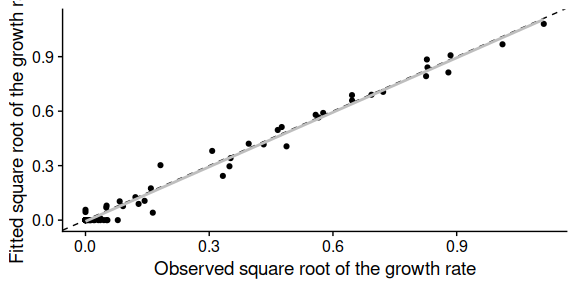In this plot, the dashed line is the line with intercept 0 and slope 1 where every point should fall in case of a perfect fit. The solid gray line is the regression line of predictions vs observations.

Alternatively, by passing the argument which=2, one can plot the observed and predicted counts as a function of the environmental factors

plot(fit_cardinal, which = 2)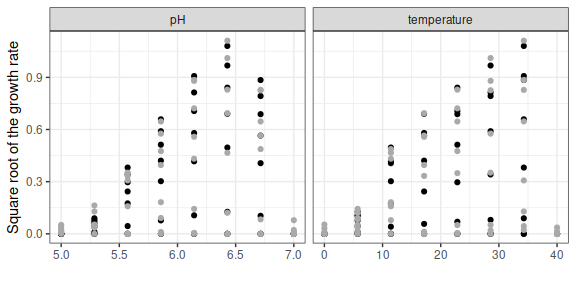A trend line can be added to this plot using the add_trend=TRUE argument:

plot(fit_cardinal, which = 2, add_trend = TRUE)
#> geom_smooth() using method = 'loess' and formula 'y ~ x'
#> geom_smooth() using method = 'loess' and formula 'y ~ x'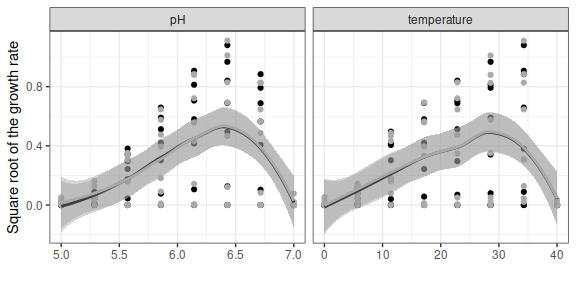Note that this line is not the predictions of the gamma model but a trend line based on the observations or the predictions. Therefore, it can be used to compare the tendency of the model predictions against the one of the observations, but it should not be used for model predictions (the predict method should be used instead).

Besides these methods, it also includes other common S3 methods for this type of object (residuals, coef, vcov, deviance, fitted, predict…). Please check the help page of FitSecondaryGrowth for a complete list of available methods.

Note that this class is a subclass of list, making it easy to access different aspects of the fit directly. Namely, it has 5 items:

• fit_results the object returned by modFit.
• secondary_model parameters of the secondary model.
• mu_opt_fit estimated growth rate under optimal storage conditions.
• data data used for the fit.
• transformation transformation applied to the growth rate before fitting.